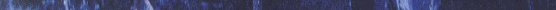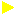# COUNTING SORT## Counting sort is a linear time sorting algorithm used to sort items when they belong to a fixed and finite set. Integers which lie in a fixed interval, say k1 to k2, are examples of such items. The algorithm proceeds by defining an ordering relation between the items from which the set to be sorted is derived (for a set of integers, this relation is trivial).Let the set to be sorted be called A. Then, an auxiliary array with size equal to the number of items in the superset is defined, say B. For each element in A, say e, the algorithm stores the number of items in A smaller than or equal to e in B(e). If the sorted set is to be stored in an array C, then for each e in A, taken in reverse order, C[B[e]] = e. After each such step, the value of B(e) is decremented. The algorithm makes two passes over A and one pass over B. If size of the range k is smaller than size of input n, then time complexity=O(n). Also, note that it is a stable algorithm, meaning that ties are resolved by reporting those elements first which occur first. This visual demonstration takes 8 randomly generated single digit numbers as input and sorts them. The range of the inputs are from 0 to 9.ANIMATIONTHE ALGORITHMApplet page